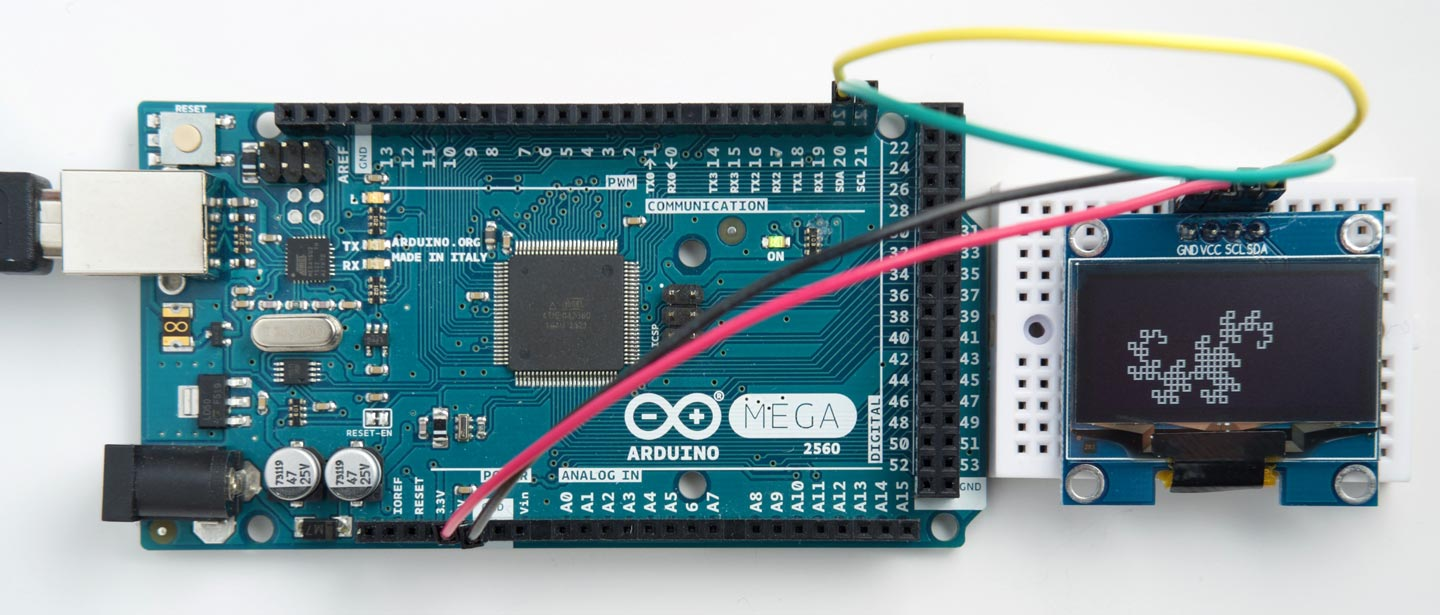## Graphics display interface in Lisp

This application shows how to interface a low-cost I2C 128x64 OLED display to an Arduino board with graphics routines written in uLisp.I've tested it with an Arduino Mega 2560 and an Arduino Due, but it should work with any board apart from the Arduino Uno, which doesn't have enough memory.

It supports I2C OLED display modules based on the SH1106 driver chip, available from a number of Chinese suppliers at a few dollars; for example, the Geekcreit 1.3" I2C OLED display, available from Banggood . Displays are available from Banggood, AliExpress, and eBay in the sizes 0.96" and 1.3" (screen diagonal), and in white, blue, or yellow/blue (the colours are fixed).

Note that this library will not work with SPI displays, or with displays based on the SSD1306 or SSD1309 driver chips, as none of these support reading back the display memory.

### The graphics commands

The library assumes the origin (0, 0) is in the bottom left corner, so the top right corner is (127, 63). It provides the following commands:

(init-display) - initialises the display.

(moveto x y) - moves the drawing position to (x, y).

(drawto x y) - draws a line from the drawing position to (x, y).

(point x y) - plots a point at (x, y).

(clear) - clears the display.

I've also included a simple Turtle Graphics application that draws a Dragon Curve, to put the display through its paces!

#### Constants

These constants are used by the routines:

```(defvar *address* 60)
(defvar *commands* #x00)
(defvar *onecommand* #x80)
(defvar *data* #x40)
(defvar *onedata* #xC0)
(defvar *init* '(#xA1 #xAF))```

#### Initialise the display

This routine sets up the display and turns it on:

```(defun init-display ()
(write-byte *commands* s)
(dolist (x *init*) (write-byte x s))))```

You need to give the (init-display) command after powering-up the display.

#### Write a command to the display

The routine single writes a single display command to the display:

```(defun single (byte s)
(write-byte *onecommand* s)
(write-byte byte s))```

#### Clear the screen

The clear routine clears the screen by writing zero bytes to the display.

```(defun clear ()
(dotimes (p 8)
(single (+ #xB0 p) s)
(dotimes (q 8)
(restart-i2c s)
(write-byte *data* s)
(dotimes (i 20) (write-byte 0 s))))))```

It's slightly complicated by the need to cater for the 32-byte buffer limit of the Arduino core I2C routines.

#### Plot a point

The point routine reads the byte from display memory, sets a bit in the appropriate position, and then writes it back again:

```(defun point (x y)
(let (j)
(single (+ #x00 (logand (+ x 2) #x0F)) s)
(single (+ #x10 (ash (+ x 2) -4)) s)
(single (+ #xB0 (ash y -3)) s)
(single #xE0 s) ; Read modify write
(write-byte *onedata* s)
(restart-i2c s 2)
(restart-i2c s)
(write-byte *onedata* s)
(write-byte (logior (ash 1 (logand y #x07)) j) s)
(single #xEE s))))```

#### Move the plotting position

The moveto routine moves the plotting position x0,y0:

```(defvar x0 0)
(defvar y0 0)

(defun moveto (x y)
(setq x0 x)
(setq y0 y))```

#### Draw a line

Finally, drawto draws from the current plotting position to the point x,y:

```(defun drawto (x y)
(let* ((dx (abs (- x x0)))
(dy (abs (- y y0)))
(sx (if (< x0 x) 1 -1))
(sy (if (< y0 y) 1 -1))
(err (- dx dy))
e2)
(loop
(point x0 y0)
(when (and (= x0 x) (= y0 y)) (return))
(setq e2 (ash err 1))
(when (> e2 (- dy)) (decf err dy) (incf x0 sx))
(when (< e2 dx) (incf err dx) (incf y0 sy)))))```

This uses Bresenham's line algorithm to draw the best line between two points without needing any divisions or multiplications .

Testing it out

At this point you should be able to enter the following commands:

```(clear)
(init-display)
(moveto 0 0)
(drawto 127 63)```

and see a nice diagonal line across the display.

I recommend you save the graphics routines into EEPROM with:

`(save-image)`

so you can load them again next time you power-up with (load-image).

### Turtle Graphics demo

Here's a simple Turtle Graphics demo which plots the recursive Dragon curve. We start with the 'turtle' at (32, 32) pointing in the positive x direction, defined by the vector v:

```(defvar x0 32)
(defvar y0 32)
(defvar v '(1 0))```

Here are the turtle commands; we only support rotations of 90° in this version:

```(defun forward (size) (drawto (+ x0 (* (first v) size)) (+ y0 (* (second v) size))))
(defun left () (setq v (list (- (second v)) (first v))))
(defun right () (setq v (list (second v) (- (first v)))))```

Finally, here's the definition of the Dragon curve:

```(defun ldragon (size level)
(cond
((= level 0) (forward size))
(t
(ldragon size (- level 1))
(left)
(rdragon size (- level 1)))))

(defun rdragon (size level)
(cond
((= level 0) (forward size))
(t
(ldragon size (- level 1))
(right)
(rdragon size (- level 1)))))```

Run the Dragon curve with the command:

`(ldragon 3 8)`

Here's the whole graphics display interface in a single file: Graphics display interface.

1. ^ Geekcreit 1.3 Inch 4 Pin White OLED LCD Display on Banggood.
2. ^ Bresenham's line algorithm on Wikipedia.

Previous: Dot-matrix clock